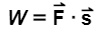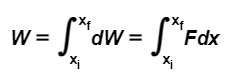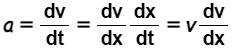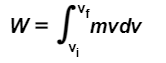# Problem: Leaming Goal: To understand the meaning and possible applications of the work-energy theorem. In this problem, you will use your prior knowledge to derive one of the most important relationships in mechanics: the work-energy theorem. We will start with a special case! a particle of mass m moving in the x-direction at constant acceleration a. During a certain interval of time, the particle accelerates from vi to vf, undergoing displacement s given by s = xf - xiPart A Find the acceleration a of the particle. Express the acceleration in terms of vi, vf, and s. Let us now consider a situation in which the particle is still moving along the x-axis, but the net force, which is still directed along the x-axis, is no longer constant. Let's see how our earlier definition of work, ,  needs to be modified by being replaced by an integral. If the path of the particle is divided into very small displacements dx, we can assume that over each of these small displacement intervals, the net force remains essentially constant and the work dW done to move the particle from x to x + dx is dW=Fdx, where F is the x component of the net force (which remains virtually constant for the small displacement from x to x + dx). The network W done on the particle is then given byNow using F = ma and Part F. Evaluate the integral:Express your answer in terms of m, vi, and vf.Part G. A particle moving in the x-direction is being acted upon by a net force F(x) = Cx2, for some constant C. The particle moves from xi = L to xf = 3L. What is ΔK, the change in kinetic energy of the particle during that time? Express your answer in terms of C and L.

###### FREE Expert Solution

From Newton's second law, the force acting on a particle:

$\overline{){\mathbf{F}}{\mathbf{=}}{\mathbf{ma}}}$

90% (95 ratings)###### Problem Details

Leaming Goal:

To understand the meaning and possible applications of the work-energy theorem. In this problem, you will use your prior knowledge to derive one of the most important relationships in mechanics: the work-energy theorem. We will start with a special case! a particle of mass m moving in the x-direction at constant acceleration a. During a certain interval of time, the particle accelerates from vi to vf, undergoing displacement s given by s = xf - xi

Part A Find the acceleration a of the particle. Express the acceleration in terms of vi, vf, and s.

Let us now consider a situation in which the particle is still moving along the x-axis, but the net force, which is still directed along the x-axis, is no longer constant. Let's see how our earlier definition of work,,  needs to be modified by being replaced by an integral. If the path of the particle is divided into very small displacements dx, we can assume that over each of these small displacement intervals, the net force remains essentially constant and the work dW done to move the particle from to x + dx is dW=Fdx, where F is the x component of the net force (which remains virtually constant for the small displacement from x to x + dx). The network W done on the particle is then given byNow using F = ma andPart F. Evaluate the integral:Express your answer in terms of m, vi, and vf.

Part G. A particle moving in the x-direction is being acted upon by a net force F(x) = Cx2, for some constant C. The particle moves from xi = L to xf = 3L. What is ΔK, the change in kinetic energy of the particle during that time?

Express your answer in terms of C and L.

Frequently Asked Questions

What scientific concept do you need to know in order to solve this problem?

Our tutors have indicated that to solve this problem you will need to apply the More Work-Energy Problems concept. You can view video lessons to learn More Work-Energy Problems. Or if you need more More Work-Energy Problems practice, you can also practice More Work-Energy Problems practice problems.

What professor is this problem relevant for?

Based on our data, we think this problem is relevant for Professor Struganova's class at UCF.